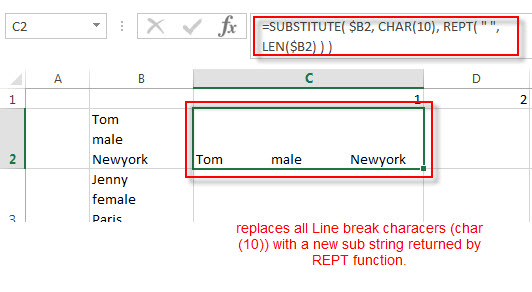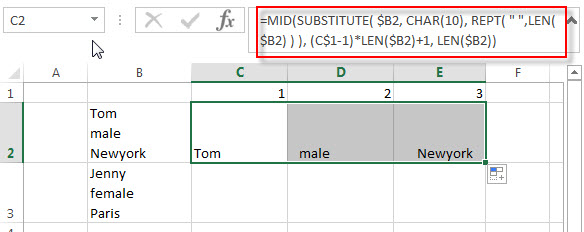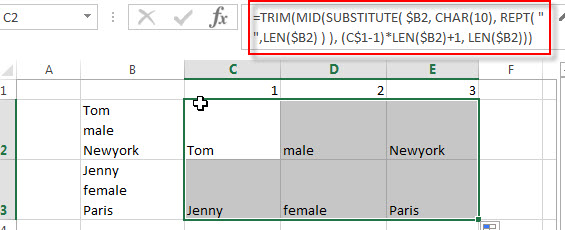# How to Split Multiple Lines from a Cell into Rows

This post will guide you how to split multiple lines from a cell into separated rows or columns in Excel. You will learn that how to extract text string separated by line break character into rows in excel 2013.

In the previous post, we talked that how to split text string by only one line break character. And this post will talk that how to split text string by multiple line break character (multiple lines) into rows.

## Split Multiple Lines from a Cell into Rows

If you have multiple lines in a cell and each line is separated by line break character or press “alt + enter” key while you entering the text string into cells, and you need to extract the multiple lines into the separated rows or columns, you can use a combination with the TRIM function, the MID function, the SUBSTITUTE function, the REPT function, the LEN function to create a complex excel formula.

For example, to extract multiple lines in Cell B2, then put it into C2, D2 or E2. You can write down the following excel formula:

`=TRIM( MID(SUBSTITUTE( \$B2, CHAR(10), REPT( " ",LEN(\$B2) ) ), (C\$1-1)*LEN(\$B2)+1, LEN(\$B2)) )`

Let’s see how this formula works:

=CHAR(10)

The CHAR function returns the character specified by a number.  If the number is 10, it will return a line break character in text string.

=LEN(\$B2)

The LEN function returns the total length of text string in Cell B2.

= REPT( ” “,LEN(\$B2)

This formula will repeat empty string a given number of times that returned by the LEN function.  It will return a new text string.

=SUBSTITUTE( \$B2, CHAR(10), REPT( ” “,LEN(\$B2) ) )The SUBSTITUTE function will replace all line break characters with a new text string returned by the REPT function in a text string in Cell B1. The returned string will go into the MID FUNCTION as its text argument.

= (C\$1-1)*LEN(\$B2)+1

This formula returns the starting position of the first, second, or third or nth substring. The returned value goes into the MID function as its start_num argument.

=MID(SUBSTITUTE( \$B2, CHAR(10), REPT( ” “,LEN(\$B2) ) ), (C\$1-1)*LEN(\$B2)+1, LEN(\$B2))The returned result of the last LEN function is the number of the characters that you want to extract from a text string in Cell B2. It goes into the MID function as its num_chars argument.

The MID function extract a substring from a text string returned by the SUBSTITUTE function based on start_num and num_chars arguments. The returned substring is what you want to extract.

=TRIM()The TRIM function removes all spaces from text string except for single spaces between words.

### Related Formulas

• Split Text String by Specified Character in Excel
you should use the Left function in combination with FIND function to split the text string in excel. And we can refer to the below formula that will find the position of “-“in Cell B1 and extract all the characters to the left of the dash character “-“.=LEFT(B1,FIND(“-“,B1,1)-1).…
• Split Text String to an Array
If you want to convert a text string into an array that split each character in text as an element, you can use an excel formula to achieve this result. the below will guide you how to use a combination of the MID function, the ROW function, the INDIRECT function and the LEN function to split a string…
• Split Text String by Line Break in Excel
When you want to split text string by line break in excel, you should use a combination of the LEFT, RIGHT, CHAR, LEN and FIND functions. The CHAR (10) function will return the line break character, the FIND function will use the returned value of the CHAR function as the first argument to locate the position of the line break character within the cell B1.…
• Split Text and Numbers
If you want to split text and numbers, you can run the following excel formula that use the MIN function, FIND function and the LEN function within the LEFT function in excel. And if you want to extract only numbers within string, then you also need to RIGHT function to combine with the above functions..…

### Related Functions

• Excel TRIM function
The Excel TRIM function removes all spaces from text string except for single spaces between words. The syntax of the TRIM function is as below:=TRIM(text)…
• Excel MID function
The Excel MID function returns a substring from a text string at the position that you specify.The syntax of the MID function is as below:= MID (text, start_num, num_chars)…
• Excel Substitute function
The Excel SUBSTITUTE function replaces a new text string for an old text string in a text string. The syntax of the SUBSTITUTE function is as below:= SUBSTITUTE  (text, old_text, new_text,[instance_num])….
• Excel CHAR function
The Excel CHAR function returns the character specified by a number (ASCII Value).The CHAR function is a build-in function in Microsoft Excel and it is categorized as a Text Function. The syntax of the CHAR function is as below:=CHAR(number)….
• Excel REPT function
The Excel REPT function repeats a text string a specified number of times.The REPT function is a build-in function in Microsoft Excel and it is categorized as a Text Function.The syntax of the REPT function is as below:= REPT  (text, number_times)….
• Excel LEN function
The Excel LEN function returns the length of a text string (the number of characters in a text string).The LEN function is a build-in function in Microsoft Excel and it is categorized as a Text Function.The syntax of the LEN function is as below:= LEN(text)…